Next: An illustrative example Up: Quantum statistics Previous: Introduction

## Symmetry requirements in quantum mechanics

Consider a gas consisting ofidentical, non-interacting, structureless particles enclosed within a container of volume. Let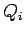denote collectively all the coordinates of the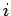th particle: i.e., the three Cartesian coordinates which determine its spatial position, as well as the spin coordinate which determines its internal state. Let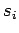be an index labeling the possible quantum states of theth particle: i.e., each possible value ofcorresponds to a specification of the three momentum components of the particle, as well as the direction of its spin orientation. According to quantum mechanics, the overall state of the system when theth particle is in state, etc., is completely determined by the complex wave-function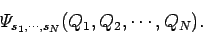(564)

In particular, the probability of an observation of the system finding theth particle with coordinates in the rangeto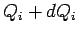, etc., is simply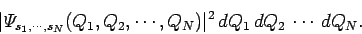(565)

One of the fundamental postulates of quantum mechanics is the essential indistinguishability of particles of the same species. What this means, in practice, is that we cannot label particles of the same species: i.e., a proton is just a proton--we cannot meaningfully talk of proton number 1 and proton number 2, etc. Note that no such constraint arises in classical mechanics. Thus, in classical mechanics particles of the same species are regarded as being distinguishable, and can, therefore, be labelled. Of course, the quantum mechanical approach is the correct one.

Suppose that we interchange theth and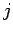th particles: i.e.,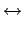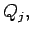(566)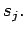(567)

If the particles are truly indistinguishable then nothing has changed: i.e., we have a particle in quantum stateand a particle in quantum state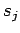both before and after the particles are swapped. Thus, the probability of observing the system in a given state also cannot have changed: i.e.,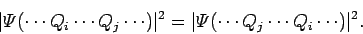(568)

Here, we have omitted the subscripts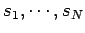for the sake of clarity. Note that we cannot conclude that the wave-function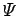is unaffected when the particles are swapped, becausecannot be observed experimentally. Only the probability density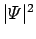is observable. Equation (568) implies that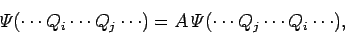(569)

whereis a complex constant of modulus unity: i.e.,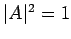.

Suppose that we interchange theth andth particles a second time. Swapping theth andth particles twice leaves the system completely unchanged: i.e., it is equivalent to doing nothing to the system. Thus, the wave-functions before and after this process must be identical. It follows from Eq. (569) that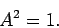(570)

Of course, the only solutions to the above equation are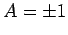.

We conclude, from the above discussion, that the wave-functionis either completely symmetric under the interchange of particles, or it is completely anti-symmetric. In other words, either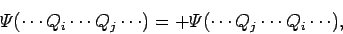(571)

or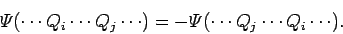(572)

In 1940 the Nobel prize winning physicist Wolfgang Pauli demonstrated, via arguments involving relativistic invariance, that the wave-function associated with a collection of identical integer-spin (i.e., spin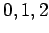, etc.) particles satisfies Eq. (571), whereas the wave-function associated with a collection of identical half-integer-spin (i.e., spin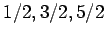, etc.) particles satisfies Eq. (572). The former type of particles are known as bosons [after the Indian physicist S.N. Bose, who first put forward Eq. (571) on empirical grounds]. The latter type of particles are called fermions (after the Italian physicists Enrico Fermi, who first studied the properties of fermion gases). Common examples of bosons are photons and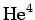atoms. Common examples of fermions are protons, neutrons, and electrons.

Consider a gas made up of identical bosons. Equation (571) implies that the interchange of any two particles does not lead to a new state of the system. Bosons must, therefore, be considered as genuinely indistinguishable when enumerating the different possible states of the gas. Note that Eq. (571) imposes no restriction on how many particles can occupy a given single-particle quantum state.

Consider a gas made up of identical fermions. Equation (572) implies that the interchange of any two particles does not lead to a new physical state of the system (sinceis invariant). Hence, fermions must also be considered genuinely indistinguishable when enumerating the different possible states of the gas. Consider the special case where particlesandlie in the same quantum state. In this case, the act of swapping the two particles is equivalent to leaving the system unchanged, so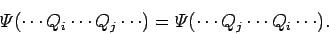(573)

However, Eq. (572) is also applicable, since the two particles are fermions. The only way in which Eqs. (572) and (573) can be reconciled is if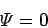(574)

wherever particlesandlie in the same quantum state. This is another way of saying that it is impossible for any two particles in a gas of fermions to lie in the same single-particle quantum state. This proposition is known as the Pauli exclusion principle, since it was first proposed by W. Pauli in 1924 on empirical grounds.

Consider, for the sake of comparison, a gas made up of identical classical particles. In this case, the particles must be considered distinguishable when enumerating the different possible states of the gas. Furthermore, there are no constraints on how many particles can occupy a given quantum state.

According to the above discussion, there are three different sets of rules which can be used to enumerate the states of a gas made up of identical particles. For a boson gas, the particles must be treated as being indistinguishable, and there is no limit to how many particles can occupy a given quantum state. This set of rules is called Bose-Einstein statistics, after S.N. Bose and A. Einstein, who first developed them. For a fermion gas, the particles must be treated as being indistinguishable, and there can never be more than one particle in any given quantum state. This set of rules is called Fermi-Dirac statistics, after E. Fermi and P.A.M. Dirac, who first developed them. Finally, for a classical gas, the particles must be treated as being distinguishable, and there is no limit to how many particles can occupy a given quantum state. This set of rules is called Maxwell-Boltzmann statistics, after J.C. Maxwell and L. Boltzmann, who first developed them.Next: An illustrative example Up: Quantum statistics Previous: Introduction
Richard Fitzpatrick 2006-02-02